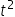Maths-
General
Easy

###Hint:

Find value offrom equation of x and then substitute in equation of y. Simplify  the equation

## Book A Free Demo+91